# Heat Transfer Networks¶

This section lists the heat networks generated by OpenModelica. The topologies are not differentiated, since OpenModelica can only create thermal networks to a limited extent. The individual pipes are mainly divided into 5 sections (sections = 5).

## Delta¶

Triangular network.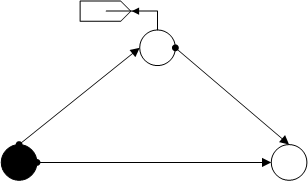heat_transfer_delta()
Returns

net - OpenModelica network converted to a pandapipes network

Return type

pandapipesNet

Example
>>> pandapipes.networks.simple_water_networks.heat_transfer_delta()


## Delta with 2 Sinks¶

Triangular network with two sinks.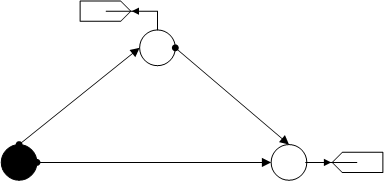heat_transfer_delta_2sinks()
Returns

net - OpenModelica network converted to a pandapipes network

Return type

pandapipesNet

Example
>>> pandapipes.networks.simple_water_networks.heat_transfer_delta_2sinks()


## Heights¶

Cross-shaped net with height differences between the individual nodes. (sections = 7, for each pipe)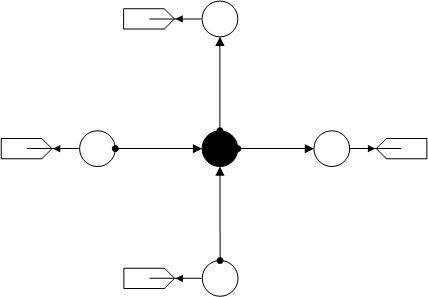heat_transfer_heights()
Returns

net - OpenModelica network converted to a pandapipes network

Return type

pandapipesNet

Example
>>> pandapipes.networks.simple_water_networks.heat_transfer_heights()


## One Pipe¶

One pipe with a sink.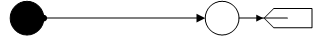heat_transfer_one_pipe()
Returns

net - OpenModelica network converted to a pandapipes network

Return type

pandapipesNet

Example
>>> pandapipes.networks.simple_water_networks.heat_transfer_one_pipe()


## One Source¶

Strand net with one source and one sink.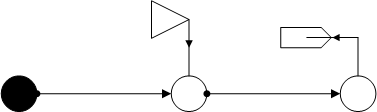heat_transfer_one_source()
Returns

net - OpenModelica network converted to a pandapipes network

Return type

pandapipesNet

Example
>>> pandapipes.networks.simple_water_networks.heat_transfer_one_source()


## Section Variation¶

Mesh network with different values for “sections” of the four pipes (4, 7, 10, 16).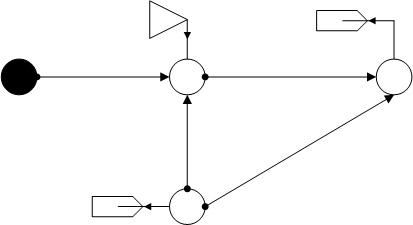heat_transfer_section_variation()
Returns

net - OpenModelica network converted to a pandapipes network

Return type

pandapipesNet

Example
>>> pandapipes.networks.simple_water_networks.heat_transfer_section_variation()


## T-Cross¶

T-cross with two sinks.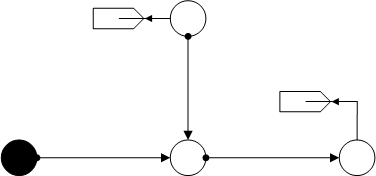heat_transfer_t_cross()
Returns

net - OpenModelica network converted to a pandapipes network

Return type

pandapipesNet

Example
>>> pandapipes.networks.simple_water_networks.heat_transfer_t_cross()


## Two Pipes¶

Strand net with two sinks.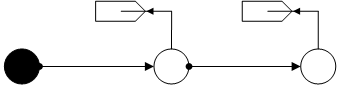heat_transfer_two_pipes()
Returns

net - OpenModelica network converted to a pandapipes network

Return type

pandapipesNet

Example
>>> pandapipes.networks.simple_water_networks.heat_transfer_two_pipes()